GFG App
Open AppBrowser
Continue

# Laspeyre’s, Paasche’s, and Fisher’s Methods of Calculating Index Number

A statistical measure that helps in finding out the percentage change in the values of different variables, such as the price of different goods, production of different goods, etc., over time is known as the Index Number. The percentage change is determined by taking a base year as a reference. This base year is the year of comparison. When an investigator studies different goods simultaneously, then the percentage change is considered the average for all the goods. There are two broad categories of Index Numbers: viz.,  Weighted Index Numbers and Unweighted Index Numbers. Weighted Index Numbers can be determined using three methods; viz., Laspeyre’s, Paasche’s, and Fisher’s Methods.

### 1. Laspeyre’s Method

The method of calculating Weighted Index Numbers under which the base year quantities are used as weights of different items is known as Laspeyre’s Method. The formula for Laspeyre’s Price Index is:Here,

P01 = Price Index of the current year

p0 = Price of goods at base year

q0 = Quantity of goods at base year

p1 = Price of goods at the current year

#### Example:

Calculate Price Index Numbers for 2021 with 2014 as base with the help of Laspeyre’s Method.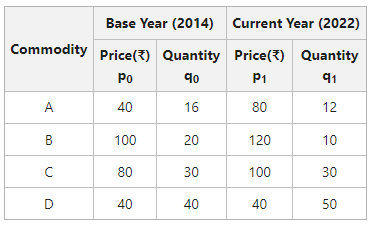#### Solution: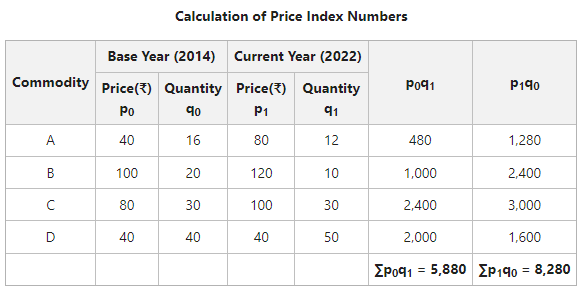= 140.81

Laspyre’s Price Index = 140.81

### 2. Paasche’s Method

The method of calculating Weighted Index Numbers under which the current year’s quantities are used as weights of different items is known as Paasche’s Method. The formula for Paasche’s Price Index is:Here,

P01 = Price Index of the current year

p0 = Price of goods in the base year

q1 = Quantity of goods in the base year

p1 = Price of goods in the current year

#### Example:

Calculate Price Index Numbers for 2021 with 2014 as base with the help of Paasche’s Method.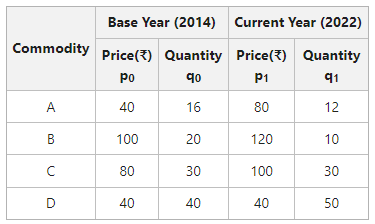#### Solution: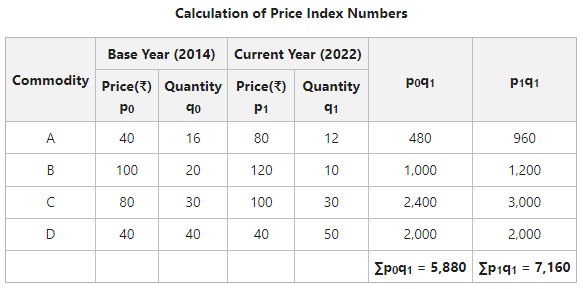= 121.76

Paasche’s Index Number = 121.76

### 3. Fisher’s Method

The method of calculating Weighted Index Numbers under which the combined techniques of Paasche and Laspeyre are used is known as Fisher’s Method. In other words, both the base year and current year’s quantities are used as weights. The formula for Fisher’s Price Index is:Here,

P01 = Price Index of the current year

p0 = Price of goods in the base year

q1 = Quantity of goods in the base year

p1 = Price of goods in the current year

Fisher’s Method is considered the Ideal Method for Constructing Index Numbers.

#### Example:

Calculate Price Index Numbers for 2021 with 2014 as the base with the help of Fisher’s Method.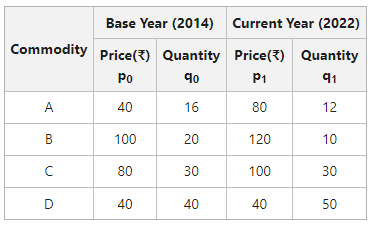#### Solution: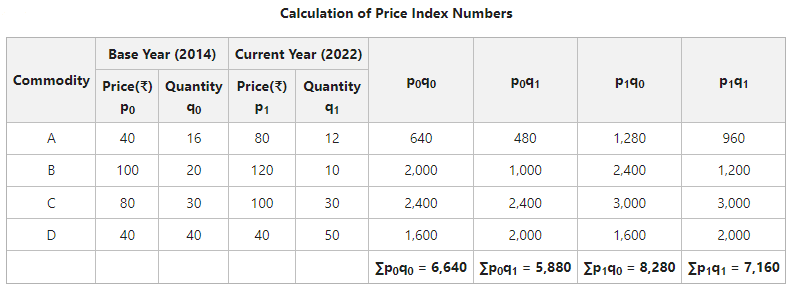= 124.45

Fisher’s Index Number = 124.45

My Personal Notes arrow_drop_up